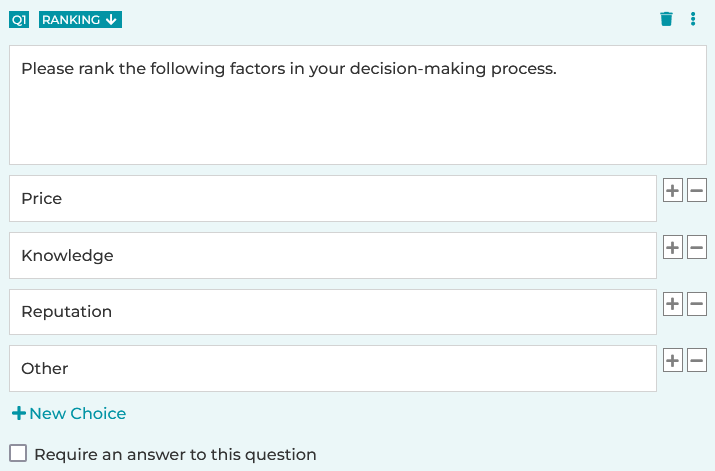# How to Create a Ranking Question

A Ranking question allows your respondents to transform a list into a ranked order, providing quantitative data to researchers like you.

1. Click on the + New Question link.
2. Choose Ranking. Alternatively, you can drag and drop your question type from the side menu to the desired position.
3. Check or uncheck the Require an answer to this question checkbox. When checked, an answer is required for the question.
3. Click on the Save button, or the Save + Add button to add a new question.

Ranking questions calculate the average ranking score for each response so you can determine which one was most preferred overall. The response with the largest average ranking score is the preferred choice.

The average ranking score is calculated as follows, where:

W = weight of ranked position
C = response count for answer choice
TC = total response count

C1W1 + C2W2 + C3W3 ... CnWn
TCWeights are applied in reverse. In other words, the respondent's most preferred choice (ranked as #1) has the largest weight, and the least preferred choice (ranked in the last position) has a weight of 1. For example, if a Ranking question has 4 answer choices, weights are assigned as follows:

•    The #1 choice has a weight of 4
•    The #2 choice has a weight of 3
•    The #3 choice has a weight of 2
•    The #4 choice has a weight of 1

Search for articles: